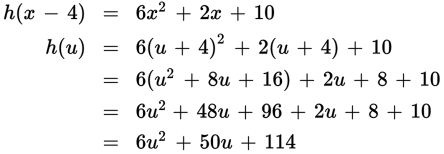# SAT Math Multiple Choice Question 420: Answer and Explanation

### Test Information

Question: 420

15. If h is a function defined over the set of all real numbers and h(x - 4) = 6x2 + 2x + 10, then which of the following defines h(x)?

• A. h(x) = 6x2 - 2x + 114
• B. h(x) = 6x2 - 46x + 98
• C. h(x) = 6x2 + 2x + 98
• D. h(x) = 6x2 + 50x + 114

Explanation:

D

Difficulty: Hard

Category: Passport to Advanced Math / Functions

Strategic Advice: The key to answering this question is in having a conceptual understanding of function notation. Here, the input (x - 4) has already been substituted and simplified in the given function. Your job is to determine what the function would have looked like had x been the input instead.

Getting to the Answer: To keep things organized, let u = x - 4, the old input. This means x = u + 4. Substitute this into h(x - 4) and simplify:This means h(u) = 6u2 + 50u + 114.

When working with function notation, you evaluate the function by substituting a given input value for the variable in the parentheses. Here, if the input value is x, then h(x) = 6x2 + 50x + 114.